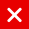# IOTA Tutorial

## Convert decimal value to balanced ternary value

In this video series different topics will be explained which will help you to understand IOTA.
It is recommended to watch each video sequentially as I may refer to certain IOTA topics explained earlier.

The trinary numeral system is often referred to as the ternary numeral system.

The ternary (or trinary) numeral system has two types:

• The balanced ternary system in which a trit has the values: -1, 0 and 1.
• The unbalanced ternary system in which a trit has the values: 0, 1 and 2.

When we speak of a base-3 numeral system we often refer to the unbalanced ternary system and not the balanced ternary system.

In a balanced ternary system, instead of using the values -1, 0 and 1 we can use other symbols, such as the letter T, 0 and 1 or the minus sign (-), 0 and the plus sign (+).
For example a balanced ternary value can be written as: 1-110-1 = 1T10T = +-+0-

When converting any base-N number to a decimal number, remember that the most left value is the most significant value and the most right value is the least significant value.

• Convert a base-2 value (binary value) to a decimal value = 1101 (bin) = 1 x 2^3 + 1 x 2^2 + 0 x 2^1 + 1 x 2^0 = 13 (dec)
• Convert a base-3 value (unbalanced ternary value) to a decimal value =2101 (ternary) = 2 x 3^3 + 1 x 3^2 + 0 x 3^1 + 1 x 3^0 = 64 (dec)
• Convert a base-10 value (decimal value) to a decimal value =6389 (dec) = 6 x 10^3 + 3 x 10^2 + 8 x 10^1 + 9 x 10^0 = 6389 (dec)
You might also like

This website uses cookies to improve your experience. We'll assume you're ok with this, but you can opt-out if you wish. AcceptRead More

Did you know?

Buy in without the stress of trying to time the market

when you setup a savings plan. If you haven’t started a plan yet, head to your Bitpanda account to get started!

Purchasing cryptocurrencies comes with a number of risks.
Never invest money that you can't afford to lose.You can automatically invest

in IOTA with Bitpanda Savings!